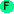# Table operations

## Table operations

Arc provides support for hash tables. An entry in the table is accessed by treating the table as a function on the key. For example, to look up `key` in table `tab`: `(tab key)`. If the key is not present, `nil` is returned. The `=` operator can be used to assign values to a table: `(= (tab key) 'value)`.

See also template operations, which provide a more complex abstraction on top of tables.obj [key value ...] Creates a table with the specified key/value pairs. (The table can be considered an object with the keys as field names.) ```>(obj key1 42 key2 "hello") #hash((key1 . 42) (key2 . "hello")) ```w/table var [body ...] Assigns an empty table to var and then executes body. ```>(w/table t1 (= (t1 "a") 42) (write-table t1)) (("a" 42)) #hash(("a" . 42)) ```tablist table Returns the table as a list of key/value pairs ```>(tablist (obj key1 42 key2 "hello")) ((key1 42) (key2 "hello")) ```listtab list Converts a list of key/value pairs to a table. ```>(listtab '((key1 val1) (key2 val2))) #hash((key1 . val1) (key2 . val2)) ```read-table [[input-port] eof] Reads a table (as a list of pairs) from the given input-port or stdin. If end-of-file is encountered, the given eof value is returned; if it is a list, it will be processed into a table. ```>(w/instring ins "((a 10)(b 20))" (read-table ins)) #hash((a . 10) (b . 20)) ```load-table filename [eof] Reads a table from the given filename. This is similar to read-table, except the input is specified as a filename, rather than an input-port. ```>load-tablet #hash((a . 10) (b . 20)) ```safe-load-table filename Loads a table from the given file, like load-table. However, if an error is encountered, an empty table is returned instead of an exception. ```>(load-table "tfile") #hash((a . 10) (b . 20)) ```load-tables filename Loads tables from the specified file until end-of-file is reached. Returns a list of tables. ```>(load-tables "tfile2") (#hash((a . 10) (b . 20)) #hash(( ```write-table h [output-port] Writes the table to the output-port (or stdout) as a list of key/value pairs. ```>(write-table (obj a 1 b 2)) ((a 1) (b 2)) nil ```save-table table filename Writes the table to the specified filename as a list of key/value pairs. ```>(save-table (obj a 10 b 20) "tfile") ((a 10) (b 20)) ```maptable proc table Applies proc to each element of the table and returns the table. ```>(maptable (fn (key val) (prn key " " val)) (obj a 1 b 2)) a 1 b 2 #hash((a . 1) (b . 2)) ```table Creates an empty hash table. ```>(table) #hash() ```fill-table table data Fills the table from the list `data`, which is interpreted as key-value pairs. `table` must already be a table. ```>(let tb (obj a 1 b 2) (fill-table tb '(c 3 d 4))) #hash((a . 1) (b . 2) (c . 3) (d . 4)) ```keys table Returns a list of keys for the table. ```>(keys (obj a 1 b 2)) (a b) ```vals table Returns a list of values for the table. ```>(vals (obj a 1 b 2)) (1 2) ```memtable keylist Creates a table with the value true for each key in `keylist`. ```>(memtable '(a b c)) #hash((a . t) (b . t) (c . t)) ```